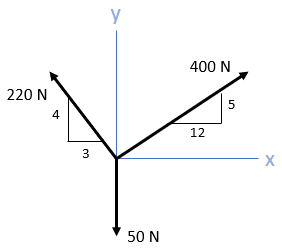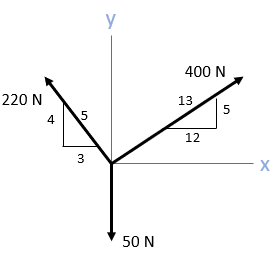## Resultant Force

In the below diagram, determine the resultant magnitude of the three forces.Hint
$$R=\sqrt{{R_{x}}^{2}+{R_{y}}^{2}}$$$Hint 2 We need to find the total forces both in the x and y directions. $$R_{x}=\sum F_{xi}$$$
$$R_{y}=\sum F_{yi}$$$$$i=A,B,C$$$
First, let's find the hypotenuse for the two given triangles/slopes for the 220 N and 400 N vectors.
$$H_{220}=\sqrt{4^2+3^2}=5$$$$$H_{400}=\sqrt{12^2+5^2}=13$$$We need to find the total forces both in the x and y directions (watch out for +/- directions!):
$$R_{x}=\sum F_{xi}$$$$$R_{y}=\sum F_{yi}$$$
$$i=A,B,C$$$$$R_{x}=-3/5(220N)+12/13(400N)+0N=-132N+369.2N=237.2N$$$
$$R_{y}=4/5(220N)+5/13⁡(400N)-50N=176N+153.8N-50=279.8N$$$Finally, to solve for the magnitude: $$R=\sqrt{{R_{x}}^{2}+{R_{y}}^{2}}=\sqrt{(237.2N)^{2}+(279.8N)^{2}}=\sqrt{134,552N^{2}}=367N$$$
367 N